Thermodynamics and Propulsion

8.6 Enhancements of, and Effect of Design Parameters on, Rankine Cycles

The basic Rankine cycle can be enhanced through processes such as superheating and reheat. Diagrams for a Rankine cycle with superheating are given in Figure 8.13. The heat addition is continued past the point of vapor saturation, in other words the vapor is heated so that its temperature is higher than the saturation temperature associated with. This does several things. First, it increases the mean temperature at which heat is added,, thus increasing the efficiency of the cycle. Second is that the quality of the two-phase mixture during the expansion is higher with superheating, so that there is less moisture content in the mixture as it flows through the turbine. (The moisture content atis less than that at.) This is an advantage in terms of decreasing the mechanical deterioration of the blading.

 [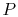-coordinates][-coordinates]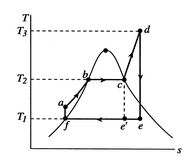[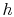-coordinates]The heat exchanges in the superheated cycle are:

• Along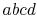, which is a constant pressure (isobaric) process: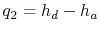.
• Along: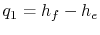,.
The thermal efficiency of the ideal Rankine cycle with superheating isThis can be expressed explicitly in terms of turbine work and compression (pump) work asCompared to the basic cycle, superheating has increased the turbine work, increased the mean temperature at which heat is received,, and increased the cycle efficiency.A comparison of the Carnot cycle and the Rankine cycle with superheating is given in Figure 8.14. The maximum and minimum temperatures are the same, but the average temperature at which heat is absorbed is lower for the Rankine cycle. To alleviate the problem of having moisture in the turbine, one can heat again after an initial expansion in a turbine, as shown in Figure 8.15, which gives a schematic of a Rankine cycle for space power application. This process is known as reheat. The main practical advantage of reheat (and of superheating) is the decrease in moisture content in the turbine because most of the heat addition in the cycle occurs in the vaporization part of the heat addition process.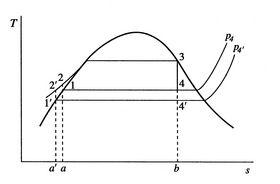We can also examine the effect of variations in design parameters on the Rankine cycle. Consider first the changes in cycle output due to a decrease in exit pressure. In terms of the cycle shown in Figure 8.16, the exit pressure would be decreased fromto. The original cycle is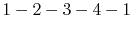, and the modified cycle is. The consequences are that the cycle work, which is the integral of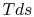around the cycle, is increased. In addition, as drawn, although the levels of the mean temperature at which the heat is absorbed and rejected both decrease, the largest change is the mean temperature of the heat rejection, so that the thermal efficiency increases.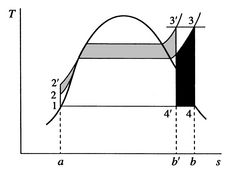Another design parameter is the maximum cycle pressure. Figure 8.17 shows a comparison of two cycles with different maximum pressure but the same maximum temperature, which is set by material properties. The average temperature at which the heat is supplied for the cycle with a higher maximum pressure is increased over the original cycle, so that the efficiency increases.

Muddy Points

Why do we look at the ratio of pump (compression) work to turbine work? We did not do that for the Brayton cycle. (MP 8.10)

Shouldn't the efficiency of the super/re-heated Rankine cycle be larger because its area is greater? (MP 8.11)

Why can't we harness the energy in the warm water after condensing the steam in a power plant? (MP 8.12)

UnifiedTP